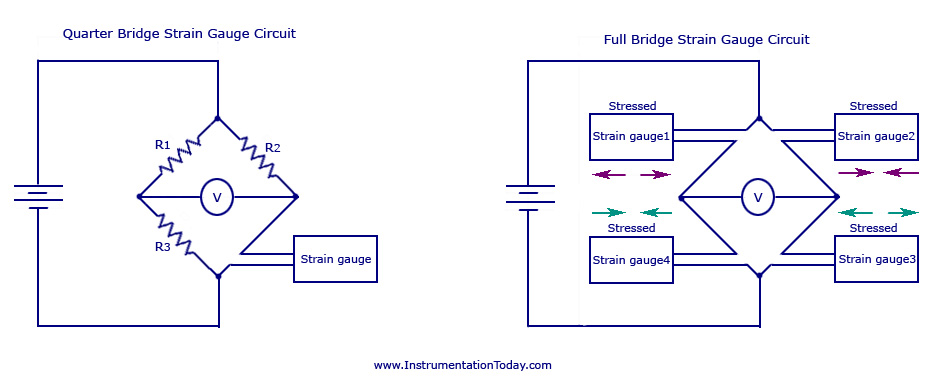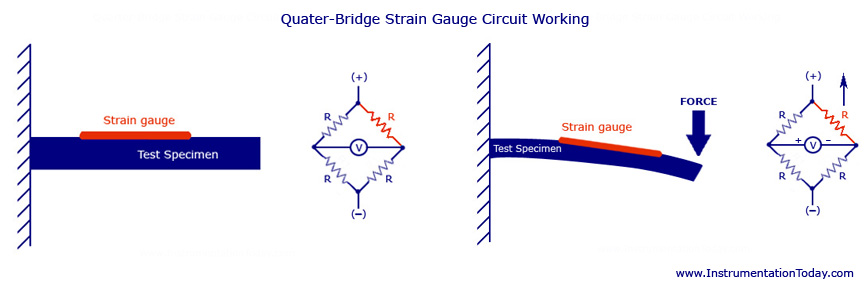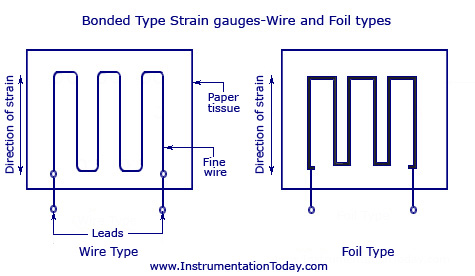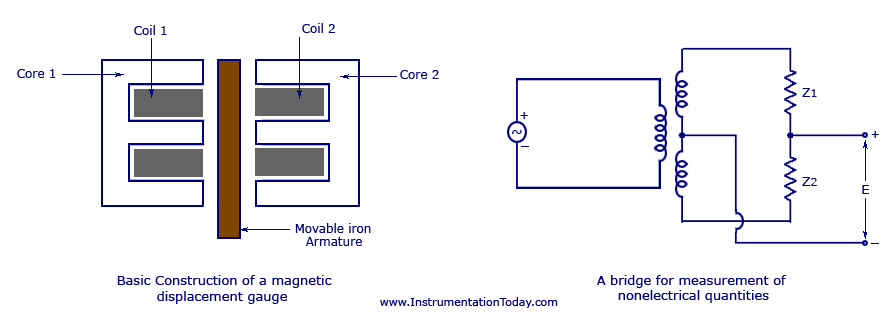UXDE dot Net Wordpress Themes

# Strain Gauge

Strain Gauge is a passive transducer that converts a mechanical elongation or displacement produced due to a force into its corresponding change in resistance R, inductance L, or capacitance C. A strain gauge is basically used to measure the strain in a work piece. If a metal piece is subjected to a tensile stress, the metal length will increase and thus will increase the electrical resistance of the material. Similarly, if the metal is subjected to compressive stress, the length will decrease, but the breadth will increase. This will also change the electrical resistance of the conductor. If both these stresses are limited within its elastic limit (the maximum limit beyond which the body fails to regain its elasticity), the metal conductor can be used to measure the amount of force given to produce the stress, through its change in resistance.

#### Strain Gauge Transducer

The device finds its wide application as a strain gauge transducer/sensor as it is very accurate in measuring the change in displacement occurred and converting it into its corresponding value of resistance, inductance or capacitance. It must be noted that the metal conductor which is subjected to an unknown force should be of finite length.

#### Types

Strain gauge transducers are broadly classified into two. They are

1. #### Electrical Resistance Type Strain Gauge

In an electrical resistance strain gauge, the device consists of a thin wire placed on a flexible paper tissue and is attached to a variety of materials to measure the strain of the material. In application, the strain gauge will be attached to a structural member with the help of special cement. The gauge position will be in such a manner that the gauge wires are aligned across the direction of the strain to be measured. The wire used for the purpose will have a diameter between 0.009 to 0.0025 centimeters. When a force is applied on the wire, there occurs a strain (consider tensile, within the elastic limit) that increases the length and decreases its area. Thus, the resistance of the wire changes. This change in resistance is proportional to the strain and is measured using a Wheatstone bridge.

A simple Wheatstone bridge circuit is shown in the figure below. It can be set in three different ways such as – full bridge, half bridge or quarter bridge. A full bridge will have all four of its gauges active. The half bridge will have two of its gauges active and thus uses two precise value resistors. The quarter bridge will have only one gauge and the rest of the resistors will be precise in value.

A full bridge circuit is used in applications where complimentary pair of strain gauges is to be bounded to the test specimen. In practice, a half bridge and full bridge circuit has more sensitivity than the quarter bridge circuit. But since, the bonding is difficult, a quarter bridge circuits are mostly used for strain gauge measurements. A full bridge circuit is said to be more linear than other circuits.

An external supply is given to the bridge as shown in the diagram. Initially, when there is no application of strain, the output measurement will be zero. Thus, the bridge is said to be balanced. With the application of a stress to the device, the bridge will become unbalanced and produces an output voltage that is proportional to the input stress.

The application of a full bridge and quarter bridge strain gauge circuit is shown in the figure below.Quarter And Full Bridge Strain Gauge Circuit

A quarter bridge output corresponding to the application of a force is shown below. Initially, the circuit will be balanced without the application of any force. When a downward force is applied, the length of the strain gauge increases and thus a change in resistance occurs. Thus an output is produced in the bridge corresponding to the strain.Quarter Bridge Strain Gauge Circuit-Working

The wire strain gauge can be further divided into two. They are bonded and unbonded strain gauge.

As shown in the figure below, an unbounded strain gauge has a resistance wire stretched between two frames. The rigid pins of the two frames are insulated. When the wire is stretched due to an applied force, there occurs a relative motion between the two frames and thus a strain is produced, causing a change in resistance value. This change of resistance value will be equal to the strain input.

A bonded strain gauge will be either a wire type or a foil type as shown in the figure below. It is connected to a paper or a thick plastic film support. The measuring leads are soldered or welded to the gauge wire. The bonded strain gauge with the paper backing is connected to the elastic member whose strain is to be measured.Bonded Type Strain Gauges

According to the strain to be measured, the gauges can be classified as the following.

• Uniaxial/Wire Strain Gauge

The figure of such a strain gauge is shown above. It mostly uses long and narrow sensing elements so as to maximize the length of the strain sensing material in the desired direction. Gauge length is chosen according to the strain to be calculated.

• Biaxial Strain Gauges

When the measurement of strain is to be done in two directions (mostly at right angles), this method is used. The basic structure for this is the two element 90 planar rosette or the 90 planar shear/stacked foil rosette. The gauges are wired in a Wheatstone bridge circuit to provide maximum output. For stress analysis, the axial and transfers elements have different resistances which can be selected that the combined output is proportional to the stress while the output of the axial element alone is proportional to the strain. The figure is given below.

• Three Element Rosettes

It is divided into two types – three element 60delta rosette strain gauge and three element 45planar rectangular rosette. They are used in applications where both the magnitude and direction of the applied strains are to be found out. Both the figures are shown below. The 60 rosette is used when the direction of the principal strain is unknown. The 45 rosette is used to determine a high angular resolution, and when the principal strains are known.

2.   Semiconductor Strain Gauge

This is the most commonly used strain gauge as a sensor, although the bonded type may also be used in stress analysis purposes. The bonded type is usually made in wafers of about 0.02 centimeters in thickness with length and resistance values nearly equal to the wire gauge. It uses either germanium or silicon base materials to be made available in both n-type or p-type. The p-type gauges have a positive gauge factor while the n-type gauges have a negative gauge factor. Temperature dependence of gauge factor is governed by the resistivity of the material. The large value of the gauge factor in semiconductor gauges is attributed to the piezoresistance effect in such materials.

#### Variable Inductance Type Strain Gauge

The basic arrangement of a variable inductance strain gauge is shown below. This type of strain gauge is very sensitive and can be used to measure small changes in length – as small as 1 millionth of an inch. Thus, it is highly applicable as a displacement transducer. The member whose strain is to be measured is connected to one end of a moveable iron armature. The long part of the armature is placed between the two cores with wires coiled in between. If the strain produced makes the armature move towards the left core (core 1), it increases the inductance of the left hand coil, that is, coil 1 and decreases the inductance of coil 2. These two coils produce the impedance Z1 and Z2 in the bridge circuit. This produces an output voltage E, which is proportional to the input displacement and hence proportional to the strain. This type of strain gauge is more accurate and sensitive than a resistive strain gauge. But, it is difficult to install the device as it is bulky and complex in construction.Variable Inductance Type Strain Gauge

#### Errors in Strain Gauge

Some of the main causes for errors and inaccuracy in the device reading are given below.

• Temperature Variation – This can be one of the major causes of error in a strain gauge. It can easily change the gauge resistance and cause differential expansion between the gauge and the test piece, causing variation in the measurable strain.
• Humidity – Humidity can affect the accuracy by the breakdown of insulation between the gauge and the ground point. It also causes electro-chemical corrosion of gauge wire due to electrolysis.
• Small errors could be caused due to thermoelectric effect.
• The gauge will be erroneous even due to small factors like zero drift, hysteresis effect and so on.
• Magnetostrictive effect can also cause errors in strain gauges of ferromagnetic materials. It produces a small voltage fluctuation of almost 2 mill volts.

#### Strain Gauge Applications### Discussion 5 Comments

1.Sherly

Nice post. Its realy nice. Many info help me.

2.danish

the information provided by instrumentationtoday is really very much beneficial…
keep it up n thans for such informations……

3.Dr.A.R.K.Murthy

An extremely useful web site to B.Tech and MSc students aswell as professors. Still some more figures are to be added for more clarification.

4.savitha

not bad

5.arunjyothi

The information which you provided is helpful to me. But applications of quarter, half and full bridges should be in points so that easy to understand